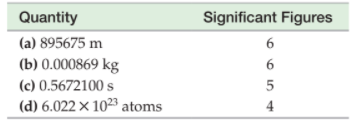×
Get Full Access to Introductory Chemistry - 5 Edition - Chapter 2 - Problem 47p
Get Full Access to Introductory Chemistry - 5 Edition - Chapter 2 - Problem 47p

×

# Correct any entries in. the table that areISBN: 9780321910295 34

## Solution for problem 47P Chapter 2

Introductory Chemistry | 5th Edition

• Textbook Solutions
• 2901 Step-by-step solutions solved by professors and subject experts
• Get 24/7 help from StudySoup virtual teaching assistantsIntroductory Chemistry | 5th Edition

4 5 1 306 Reviews
29
1
Problem 47P

Correct any entries in the table that are wrong.Step-by-Step Solution:
Step 1 of 3

Solution: Here, we are going to identify the wrong entry of the significant figures in the given table.

Step1:

The following rules are followed to count the number of significant figures in a particular number:

1. All non-zero digits are significant.
2. Zeroes between two non zero digits are significant.
3. The zeroes preceding to the first non zero number(i.e., to the left of the first non zero number) are not significant.
4. All zeroes placed at the end or to the right of a number are significant provided they are on the right side of the decimal point.
5. In exponential notations, the numerical portion represents the number of significant figures.

Step2:

Based on the rules given above, we can say that two of the entries given in the table are wrong.

b)        0.00086 has two significant figures        [Rule 3]

c)        0.5672100 has seven significant figures        [Rule 4]

------------------

Step 2 of 3

Step 3 of 3

##### ISBN: 9780321910295

This textbook survival guide was created for the textbook: Introductory Chemistry, edition: 5. Since the solution to 47P from 2 chapter was answered, more than 653 students have viewed the full step-by-step answer. The answer to “?Correct any entries in the table that are wrong.” is broken down into a number of easy to follow steps, and 9 words. This full solution covers the following key subjects: any, atoms, correct, entries, figures. This expansive textbook survival guide covers 19 chapters, and 2046 solutions. The full step-by-step solution to problem: 47P from chapter: 2 was answered by , our top Chemistry solution expert on 05/06/17, 06:45PM. Introductory Chemistry was written by and is associated to the ISBN: 9780321910295.

## Discover and learn what students are asking

Statistics: Informed Decisions Using Data : Inference about the Difference between Two Medians: Dependent Samples
?In Problems 3–10, use the Wilcoxon matched-pairs signedranks test to test the given hypotheses at the a = 0.05 level of significance. The dependent sa

Unlock Textbook Solution